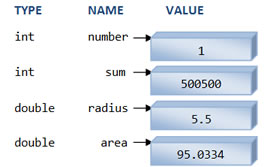# Difference between Integer and Double

##### Key Difference: In programming languages, integer and double are both data types (arithmetic type specifiers) used for the definition of a variable before it is used. Integer is used as a data type to denote an integer number, whereas double is a data type to denote a big floating number.Programming languages consist of various types of data types like int, float, double, etc. Integers can be generally described as types of whole numbers that are not in a form of fraction. Integers can be both positive and negative. Integers are actually subset of real numbers. It is important to mention that 0 is also included in the list of integers. In programming languages, int is used to define an integer number. Integers are stored in various sizes.

They can be signed or unsigned. In the code below, number is an integer and is defined by using a keyword int. In Java, the group of integers includes byte, short, int, and long, which are for signed and unsigned numbers including zero.

{

int Count;

Count = 5;

}

For floating point numbers, the programming languages are generally equipped with two basic data types known as float and double. Float is generally used to define small floating point numbers, whereas double is used to define big floating numbers. A double provides an approximate precision of 14 decimal digits. Typically, it is stored in 8 bytes with 56 bits of signed mantissa and 8 bits of signed exponent. Both int and double are used with modifiers like short, long, signed and unsigned. The types float, double and the variant long double, are all considered to be floating-point types.

{

double atoms;

atoms = 2304.01;

}

Comparison between Double and Integer in C programming language:

 Double Integer Definition It is used as a data type to denote big floating numbers. It is used as a data type to denote an integer number. Keyword to define double int Type Double Long double Short int Unsigned short int Unsigned int Int Long int Bytes Double - 8 Long double - 12 Short int - 2 Unsigned short int - 2 Unsigned int - 4 Int - 4 Long int - 4 Bits Double - 64 Long double - 96 Short int - 16 Unsigned short int - 16 Unsigned int - 16 Int - 32 Long int - 32

Image Courtesy: ntu.edu.sg